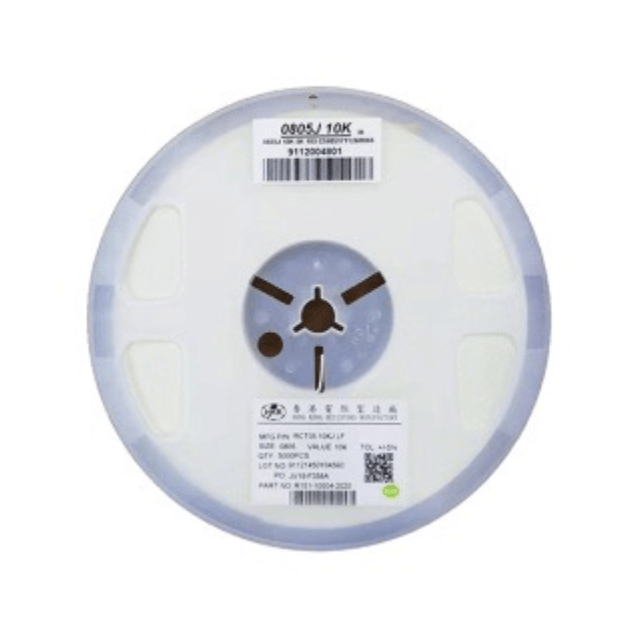English/ / / Thick Film Chip ResistorShare to:

# Thick Film Chip Resistor

model:0201
color:pink• 0201

Packing：Tape on reel

Tolerance：±1%, ±5%

Resistance Value Range：1 OHM - 100 MEG OHM

HKR products comply with environmental requirement in accordance with SONY of code no. SS-00259 for not related in 8 harmful materials.

· Small size and ligtweight with size range per int'l standard

· Highly stable in auto-placement surface mounting application

· Compatible with both wave soldering and reflow soldering

Configuration

Dimensions

Power Derating Curve

RCT Series

The working voltage is calculated based on the resistance value following the formula of V=√(P*R) or to its maximum extent as indicated above.

The overload voltage is calculated based on the resistance value following the formula of V=2.5√(P*R) or to i maximum extent as indicated above.

Soldering Temperature Curve

Specification And Test Methods

Markings

1.1 No markings on 0201 and 0402. Markings on the other sizes are expressed by a 3-digit code in its exact value

E. G. ： 6R8=6.8;100=10×10 0=10;472=47×102=4700=4.7K

1.2.+/-1% Resistance value markings

a. Markings on sizes 0805, 1206 and above are expressed by a 4-digit code.

E. G. ：82R5=82.5;1000=100×10 0=100;2212=221×102=22100=22.1K。

b. Markings on 0603 ±1%( IEC E–96 Series ) are expressed by a 3-digit code; the first two digits represent the value code and the last capital letter represents the multiplier.

The coding system of the E-96 series is as follows :

Multiplier code：

E. G. ：01Y=100× 10 -2=1;68A=499×100=499;02D=102×103=102K

c. Any resistance values of 1% tolerance but not included in the E-96 series, the value markings are the same as +/-5% tolerance with labelling identification.

d. Marking of the 0 Ohm resistor is a '0' with its value range from 0-50mΩ. No tolerance shown on the product label.

Tape And Reel Package

Outer Packaging

the first package：1~10 reels the second package：66 reels Max

· When quantity shall not reach the max, the remaining empty space shall be buried with buffer material.

· When the quantity is a few, altemative packing methods may be used. It is important to ensure the safety of the products during transportation.

Packing：Tape on reel

Tolerance：±1%, ±5%

Resistance Value Range：1 OHM - 100 MEG OHM

HKR products comply with environmental requirement in accordance with SONY of code no. SS-00259 for not related in 8 harmful materials.

· Small size and ligtweight with size range per int'l standard

· Highly stable in auto-placement surface mounting application

· Compatible with both wave soldering and reflow soldering

Configuration

Dimensions

Power Derating Curve

RCT Series

The working voltage is calculated based on the resistance value following the formula of V=√(P*R) or to its maximum extent as indicated above.

The overload voltage is calculated based on the resistance value following the formula of V=2.5√(P*R) or to i maximum extent as indicated above.

Soldering Temperature Curve

Specification And Test Methods

Markings

1.1 No markings on 0201 and 0402. Markings on the other sizes are expressed by a 3-digit code in its exact value

E. G. ： 6R8=6.8;100=10×10 0=10;472=47×102=4700=4.7K

1.2.+/-1% Resistance value markings

a. Markings on sizes 0805, 1206 and above are expressed by a 4-digit code.

E. G. ：82R5=82.5;1000=100×10 0=100;2212=221×102=22100=22.1K。

b. Markings on 0603 ±1%( IEC E–96 Series ) are expressed by a 3-digit code; the first two digits represent the value code and the last capital letter represents the multiplier.

The coding system of the E-96 series is as follows :

Multiplier code：

E. G. ：01Y=100× 10 -2=1;68A=499×100=499;02D=102×103=102K

c. Any resistance values of 1% tolerance but not included in the E-96 series, the value markings are the same as +/-5% tolerance with labelling identification.

d. Marking of the 0 Ohm resistor is a '0' with its value range from 0-50mΩ. No tolerance shown on the product label.

Tape And Reel Package

Outer Packaging

the first package：1~10 reels the second package：66 reels Max

· When quantity shall not reach the max, the remaining empty space shall be buried with buffer material.

· When the quantity is a few, altemative packing methods may be used. It is important to ensure the safety of the products during transportation.

Previous:
Next:
Hong Kong Resistors Manufactory
Resistor Is Our Name